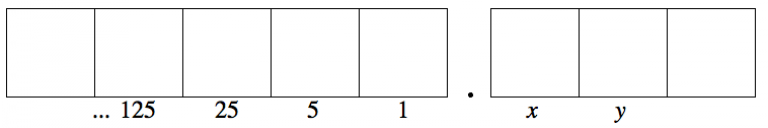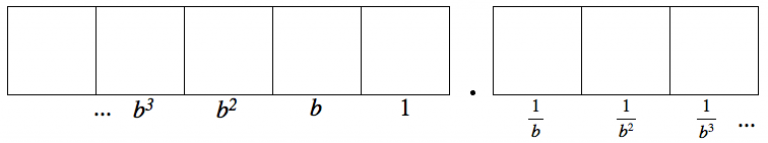# 7.3: x-mals

$$\newcommand{\vecs}{\overset { \rightharpoonup} {\mathbf{#1}} }$$ $$\newcommand{\vecd}{\overset{-\!-\!\rightharpoonup}{\vphantom{a}\smash {#1}}}$$$$\newcommand{\id}{\mathrm{id}}$$ $$\newcommand{\Span}{\mathrm{span}}$$ $$\newcommand{\kernel}{\mathrm{null}\,}$$ $$\newcommand{\range}{\mathrm{range}\,}$$ $$\newcommand{\RealPart}{\mathrm{Re}}$$ $$\newcommand{\ImaginaryPart}{\mathrm{Im}}$$ $$\newcommand{\Argument}{\mathrm{Arg}}$$ $$\newcommand{\norm}{\| #1 \|}$$ $$\newcommand{\inner}{\langle #1, #2 \rangle}$$ $$\newcommand{\Span}{\mathrm{span}}$$ $$\newcommand{\id}{\mathrm{id}}$$ $$\newcommand{\Span}{\mathrm{span}}$$ $$\newcommand{\kernel}{\mathrm{null}\,}$$ $$\newcommand{\range}{\mathrm{range}\,}$$ $$\newcommand{\RealPart}{\mathrm{Re}}$$ $$\newcommand{\ImaginaryPart}{\mathrm{Im}}$$ $$\newcommand{\Argument}{\mathrm{Arg}}$$ $$\newcommand{\norm}{\| #1 \|}$$ $$\newcommand{\inner}{\langle #1, #2 \rangle}$$ $$\newcommand{\Span}{\mathrm{span}}$$$$\newcommand{\AA}{\unicode[.8,0]{x212B}}$$

Just like in base 10, we can add boxes to the right of the decimal point in other bases, like base 5.However, the prefix “dec” in “decimal point” means ten. So we really shouldn’t call it a decimal point anymore. Maybe a “pentimal point”? (In fact, the general term is radix point.)

##### Think / Pair / Share
• Use reasoning like you saw on page 6 for the base ten system to think about other number systems:
• Figure out the values of x and y in the picture of the base-5 system above. Be sure you can explain your reasoning.
• Draw a base-4 “Dots & Boxes” model, including a radix point and some boxes to the right. Label at least three boxes to the left of the ones place and three boxes to the right of the ones place.
• Draw a base-6 “Dots & Boxes” model, including a radix point and some boxes to the right. Label at least three boxes to the left of the ones place and three boxes to the right of the ones place

In general, in a base-b system, the boxes to the left of the ones place represent positive powers of the base b. Boxes to the right of the ones place represent reciprocals of those powers.Work on the following exercises on your own or with a partner.

1. Draw a “Dots & Boxes” picture of each number. $$(a)\; 0.03_{five} \quad (b)\; 0.22_{six} \quad (c)\; 0.103_{four} \quad (d)\; 0.002_{three} \ldotp$$
2. Find a familiar (base-10) fraction value for each number. $$(a)\; 0.04_{five} \quad (b)\; 0.3_{six} \quad (c)\; 0.02_{four} \quad (d)\; 0.03_{nine} \ldotp$$
3. Find a familiar (base-10) fraction value for each number. (You might want to re-read the example of $$0.31$$ in base ten from the previous section.) $$(a)\; 0.13_{five} \quad (b)\; 0.25_{six} \quad (c)\; 0.101_{two} \quad (d)\; 0.24_{seven} \quad (e)\; 0.55_{eight} \ldotp$$
##### Think / Pair / Share

Tami and Courtney were working on converting $$0.44_{five}$$ to a familiar base-10 fraction. Courtney said this:

The places in base five to the right of the point are like $$\frac{1}{5}$$ and then $$\frac{1}{25}$$. Since this has two places, the answer should be $$\frac{44}{25}$$.Tami thought about what Courtney said and replied:

I don’t know what the right answer is, but I know that can’t be right. The number $$0.44_{five}$$ is less than one, since there are no numbers in the ones place and no explosions that we can do. But the fraction $$\frac{44}{25}$$ is more than one. It’s almost two. So they can’t be the same number.

• Who makes the most sense, Courtney or Tami? Why do you think so?
• Find the right answer to the problem Courtney and Tami were working on.
##### Problem 1

Find the “decimal” representation of $$\frac{1}{4}$$ in each of the following bases. Be sure that you can justify your answer. (You might want to review the example of $$12 \frac{3}{4}$$ in the previous section.) $\begin{split} base\; 2 \qquad base\; 4& \qquad base\; 6 \\ base\; 8 \qquad base\; 10& \qquad base\; 12 \end{split} \nonumber$

This page titled 7.3: x-mals is shared under a CC BY-SA 4.0 license and was authored, remixed, and/or curated by Michelle Manes via source content that was edited to the style and standards of the LibreTexts platform; a detailed edit history is available upon request.